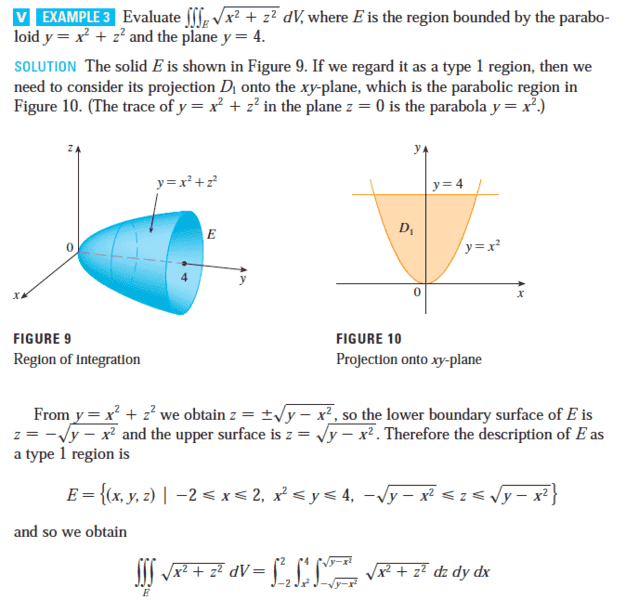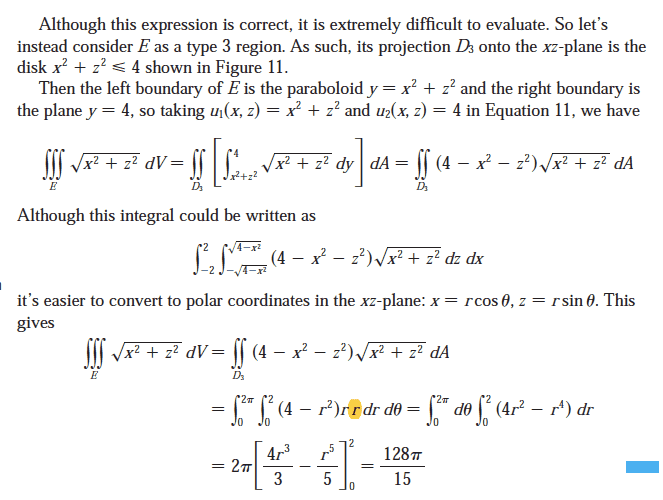# Triple integration - find volume

Gold Member

## Homework Statementin the pic

## The Attempt at a Solution

why is there an extra "r" in the highlighted line?

my attempt:
## \int_0^{2\pi}\int_0^2\int_{r^2}^41\ dy\ r\ dr\ d\theta ##
= ##\int_0^{2\pi}\int_0^2\left(4-r^2\right)\ r\ dr\ d\theta##

thanks

#### Attachments

why is there an extra "r" in the highlighted line?
Because it is part of the correct form of the differential area in polar coordinates.
$$dA=r\;dr\;d\theta$$

•MarekKuzmicki
Gold Member
##\int_0^{2\pi}\int_0^2\int_{r^2}^41\ dy\ r\ dr\ d\theta##
I have considered r dr dθ already in my calculation... but the answer is r *r *dr*dθ

Mentor
I have considered r dr dθ already in my calculation... but the answer is r *r *dr*dθ
It comes from the substitutions for polar coordinates in the x-z plane, for the expression ##\sqrt{x^2 + z^2}##. This is explained in the example where they use ##x = r\cos(\theta)## and ##z = r\sin(\theta)##.

Gold Member
√x^2+z
but why is there exists this expression in the first line of the second pic?
isn't that always 1 in the middle and integrate by looking at the boundaries for the triple integration?

my attempt : ##\int_0^{2\pi}\int_0^2\int_{r^2}^41\ dy\ r\ dr\ dθ##
mechanism: I would like to get the height first, then get the area of the cylinder

(my concepts are a little bit messy... sorry for that... and thank you very much for your patience)

Mentor
but why is there exists this expression in the first line of the second pic?
isn't that always 1 in the middle and integrate by looking at the boundaries for the triple integration?
my attempt : ##\int_0^{2\pi}\int_0^2\int_{r^2}^41\ dy\ r\ dr\ dθ##

(my concepts are a little bit messy... sorry for that... and thank you very much for your patience)
If the goal is to find the volume using a triple integral, then the integrand will be 1, something like this ##\iiint_E 1~dV##.
However, in this problem you are asked to evaluate an integral that doesn't represent a volume.

Gold Member
the solution: ## \int_0^{2\pi}\int_0^2\int_{r^2}^4r\ dy\ r\ dr\ dθ ##
so why the solution put r in the middle?

Mentor
the solution: ## \int_0^{2\pi}\int_0^2\int_{r^2}^4r\ dy\ r\ dr\ dθ ##
so why the solution put r in the middle?
I don't know what you're talking about. The solution in the example you posted doesn't have y in it. They converted the integral to polar form - no x, y, or z.

Gold Member
I don't know what you're talking about. The solution in the example you posted doesn't have y in it. They converted the integral to polar form - no x, y, or z.
its the same...
##\int_0^{2\pi}\int_0^2\int_{r^2}^4r\ dy\ r\ dr\ dθ## =## \int_0^{2\pi}\int_0^2\left(4-r^2\right)r\ \ r\ dr\ dθ##

so why the solution put r in the middle?

Mentor
its the same...
##\int_0^{2\pi}\int_0^2\int_{r^2}^4r\ dy\ r\ dr\ dθ## =## \int_0^{2\pi}\int_0^2\left(4-r^2\right)r\ \ r\ dr\ dθ##

so why the solution put r in the middle?
I don't know. It seems that you put it in the middle, since the textbook image you posted doesn't have this integral in it - ##\int_0^{2\pi}\int_0^2\int_{r^2}^4r\ dy\ r\ dr\ dθ##.

You could rewrite that integral like so: ##\int_0^{2\pi}\int_0^2\int_{r^2}^4\ dy\ r^2\ dr\ dθ##, and it wouldn't make any difference - you get the same integral as shown on the right in what I quoted from you.

Gold Member
But why not## \int_0^{2\pi}\int_0^2\int_{r^2}^41\ dy\ r\ dr\ dθ## which is 1 in the middle?
why is solution is ##\int_0^{2\pi}\int_0^2\int_{r^2}^4r\ dy\ r\ dr\ dθ## = ##\int_0^{2\pi}\int_0^2\left(4-r^2\right)r\ \ r\ dr\ dθ## ?

However, in this problem you are asked to evaluate an integral that doesn't represent a volume
it is asking for volume in the question...

Mentor
But why not## \int_0^{2\pi}\int_0^2\int_{r^2}^41\ dy\ r\ dr\ dθ## which is 1 in the middle?
You keep asking this question, which was answered in posts #2 and #4. What parts of those posts do you not understand?
yecko said:
why is solution is ##\int_0^{2\pi}\int_0^2\int_{r^2}^4r\ dy\ r\ dr\ dθ## = ##\int_0^{2\pi}\int_0^2\left(4-r^2\right)r\ \ r\ dr\ dθ## ?

it is asking for volume in the question...
NO, IT IS NOT!
As it says in the image you posted,
Evaluate ##\iiint_E \sqrt{x^2 + z^2}~dV##, where E is the region bounded by the paraboloid ##y = x^2 + z^2## and the plane ##y = 4##.
This is NOT a volume integral!